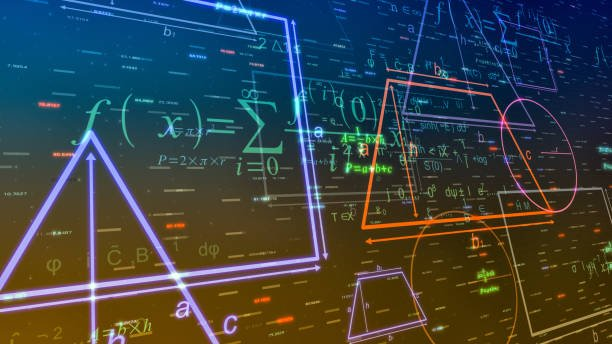## Applications of Linear Maps

In mathematics, there exist several types of maps. Linear maps are some of these maps found in this field. Linear mapping is the operation of mapping vector spaces that preserve scalar multiplication and vector addition. For example, let us assume we have two vector spaces, A and B, and a field, K. Then, we can perform mapping to these vectors to have f: A->B, which is the linear map in this case. This article will discuss some typical applications of linear mapping in mathematics.

1. Geometric Transformation

Transformation works hand-in-hand with linear maps. In transformation, geometrics objects are manipulated, and their shape and size are altered. Linear-mapping knowledge is extensively used to generate linear transformation maps to achieve this.2. Computer Graphics

Linear maps are crucial in computer graphics. In graphics, the knowledge of linear mapping helps define how the images are mapped onto the screen. Transformation matrices change the graphics’ position, size, and orientation.

3. Reflections

In reflection, a linear mapping technique is applied. Here, the linear mapping knowledge affects points on the plane or an XY-plane to its mirror-like image depending on the transformation matrix. In geometric, linear mapping can cause reflection on the x-axis, y-axis, and y=x axis.

4. Shear mapping

Shear mapping is a branch in plane geometry that applies the knowledge of linear mapping in displacing points to a given direction. Linear maps can produce two types of shear maps; the horizontal shear map and the vertical shear map. The linear mapping technique displaces the points to the right in horizontal shear. On the other side, in the vertical shearing, points are displaced upwards.

5. Compiler Optimizations

Linear map functions are essential in generating the transformed loop. The technique helps minimize some attributes in the nested loop, increasing the program’s performance.

6. Mapping Vector spaces

Linear mapping is used in mapping vector spaces that are addictive and multiplicative. In this context, the technique takes two inputs (vector spaces) over a given field and outputs a linear map. For example, let’s say we have Vectors K and M over field P. Then, our linear map would be:

F: K->M where f is the function.

7. Parallelizing Compilers

Parallelizing compiler is an operation generating parallelism in a sequential program. Numerous ways can be used to do this, but linear mapping is the best method.

8. Rotation

Rotation is a linear mapping operation that involves rotating points about an axis. Here you can rotate the issues in a clockwise or anticlockwise direction depending on your chosen transformation matrix.

9. Scaling of 3D or 2D objects

Scaling is the process of altering the size of a given object. In Graphics and other geometric-related fields, the technique of linear mapping plays a vital role in scaling the size of things.

10. Describing change

The linear mapping technique is used to describe changes. In calculus, linear mapping tracks the transformations of derivatives and relativity.

This site uses Akismet to reduce spam. Learn how your comment data is processed.